Editor's Note: This article is a two part series about the design of anechoic chambers giving a thorough treatment of the subject that could be used as a reference piece. Part one covers RF absorber approximations for rectangular far field ranges. Part two will cover compact and near field ranges and will be published in the February 2016 issue.

The task of adequately specifying performance for an indoor anechoic chamber without driving unnecessary costs or specifying contradictory requirements calls for insight that is not always available to the author of the specification. While there are some articles and books1-3 that address anechoic chamber design, a concise compendium of reference information and rules of thumb on the subject would be useful. This article intends to be a helpful tool in that regard. It starts by recommending the proper type of range for different antenna types and frequencies of operation. Rules of thumb are provided to select the best approach for the required test or antenna type. The article concentrates on rectangular chambers. Simple approximations are used for absorber performance to generate a series of charts that can be used as a guide to specify performance and appropriate facility size.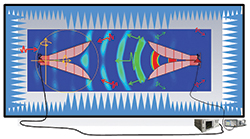Figure 1 General geometry of an indoor range - two antennas are located in the range (one for transmitting and one for receiving).

The ability to measure an antenna is an important design requirement in determining if energy is radiating properly and in the desired direction, as well as how much energy is traveling in undesired directions. To measure antennas (like many other devices that are being measured), there is the desire to have the antenna unaffected by its surrounding environment. This is where the anechoic chamber becomes a viable solution. The anechoic chamber provides an environment free of echo or other radiated signals to reduce the effects of these undesirable signals.

This article covers applications where an antenna is radiating or receiving a given signal, and its performance as a function of direction is being measured.

Range Type Selection

The general range geometry is shown in Figure 1. There are several methods of measuring the radiation patterns of antennas indoors: far field illumination, near field measurements and compact range. While they all present pros and cons, there is not a single solution that is ideal for all types of antennas and situations. The type of range most suitable for a given type of antenna is driven by two parameters: frequency and electrical size of the antenna under test (AUT). The far field condition given by the following equation drives the selection:Figure 2 The far field distance plotted related to the wavelength.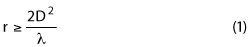The parameters mentioned above are embedded into the far field equation. D is the largest physical dimension of the antenna. Wavelength is λ, which is related to the frequency of operation on the antenna. For smaller antennas the far field range length, r, can be approximated by:4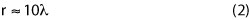This equation can be used when the antenna is under one wavelength in electrical size. From Equation 1, the far field distance can be plotted as a function of the electrical size of the antenna, as shown in Figure 2.

As a rule of thumb for indoor ranges, the far field illumination techniques are better suited for antenna sizes under 10λ. This rule is related to the electrical antenna size. Frequency of operation adds another factor that will influence the type of range. An antenna with a size of 10λ will have a far field distance of 200λ, making the test distance 20 times the size of the antenna. At some microwave frequencies this may be a test distance of 200 inches (5 m) so an indoor range may be easy to implement. However, note a 20λ antenna will have a test distance that is 800λ.

For example, consider an 18 inch dish used by a popular satellite TV service. This satellite service operates at 18.55 GHz. The dish antenna is 28.29λ in size. The far field is at approximately 1600λ or 25.86 m (84.84 ft). Clearly, for such an electrically large antenna, a far field illumination approach indoors is not economically feasible. For this antenna, a compact range or a near field approach is more suitable. Conversely, a 10λ antenna at 300 MHz, which is 10 m in size, would be extremely difficult to manipulate at a test distance of 200 m. For this case, the best solution would be an outdoor range.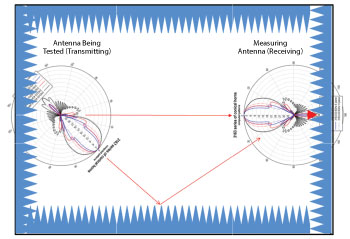Figure 3 An indoor range showing one of the reflected paths and the direct path between the AUT and the source antennas.

In general, for frequencies below 100 MHz, an outdoor range is a better approach. Current absorber technology does not support some indoor measurements at those low frequencies. Indoor ranges can be built, but the antenna size should be kept less than 2λ; which limits the far field distance to 8λ (24 m). This distance is close to the 10λ given by Equation 2. Table 1 provides an approximate guide for the different antenna sizes and frequencies of operation.

The values in Table 1 are general guidelines. Spherical near field (SNF) ranges can test antennas as small as λ/2. But for such a small antenna it may be a better approach to use a far field illumination range as it relates to the typical electrical size of the AUT.

When creating an anechoic chamber, the goal is to obtain a volume in the chamber where any reflected energy from the walls of the range (ceiling and floor) will be much lower than any of the features of interest on the radiation pattern. This volume is known as the quiet zone (QZ). Figure 1 shows that as one antenna transmits, it illuminates the receive antenna and all the walls and surfaces of the range. The energy incident onto these surfaces will be reflected towards the QZ.1,3 The level of reflected energy must be a given number of decibels below the direct path between the transmitting and receiving antennas.

As the antenna being measured is rotated (see Figure 3), its main beam will illuminate different surfaces of the chamber. The range antenna will measure the level of field radiated by the AUT along the direct path between the two antennas. However, the range antenna will also receive the reflected energy from the walls, ceiling and floor. If the reflected energy level is higher than the energy radiated along the direct path between the two antennas, then the radiation pattern in that direction cannot be measured accurately. In Figure 3, the measuring antenna, (also known as range antenna or source antenna) is pointing at a null, but it is also receiving the reflected signal from the wall that is illuminated by the main beam of the AUT. The range antenna is receiving the reflected signal in a direction of 30°. In that 30° direction, the gain of the range is lower than in the direct path (boresight) to the AUT. The reflected energy is a number of dB lower, for example, 20 dB. Let us assume that the gain in the 30° direction is 10 dB lower than the boresight. The signal received by the antenna on that direction will be -30 dB compared to the energy received when the main beam of the AUT was pointing to the range antenna. If the null is less than -30 dB, the measured pattern will have errors.5

RF Absorber

A key design item for an anechoic chamber is the RF absorber. The absorber treatment must be such that the reflected energy has a small or negligible effect on the measured data. A typical RF absorber is a lossy material shaped to allow for incoming electromagnetic waves to penetrate with minimal reflections. Once the electromagnetic (EM) energy travels inside the material, the RF energy transforms into thermal energy and dissipates into the surrounding air.6 The electrical thickness of the material determines how much energy is absorbed. The reflection level at normal incidence can be approximated by the following equation:where t is the thickness in wavelengths. The equation is valid for 0.25 ≤ t ≤ 20. This approximation can be used to get a conservative reflectivity value of an absorber of a given thickness. Most manufacturers provide the information in their datasheets.

Figure 1 shows that some of the absorber in the range is not located in the normal incident wave direction, but rather in an oblique incidence. For oblique incidence, the main reflectivity of the absorber is in the bi-static direction. Backscattering occurs when the distance between the tips of the pyramids is ≥λ.7 Hemming1 provides plots that show the estimated bi-static reflectivity of absorber at oblique incidence. A series of polynomial approximations, together with Equation 3, provide a general description of the performance of pyramidal absorbers of different thicknesses and at different angles of incidence. These are conservative approximations. That leaves a margin of error to account for things like lights, doors, positioning equipment and edge diffractions from treatment discontinuities.

The absorber performance in dB is given by the following polynomial:The coefficients in this equation are functions of the thickness. When the thickness of the absorber is such that 0.25λ ≤ t ≤ 2λ, the coefficients of Equation 4 are given by the following polynominals:When the thickness of the treatment is such that 2λ ≤ t ≤ 20λ, then the coefficients are given by the set of polynominals: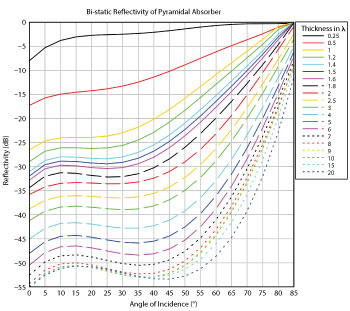Figure 4 Estimated reflectivity of RF absorber as a function of angle of incidence.

The domain of Equation 4 is limited by those angles of incidence where 0° ≤ θ ≤ 85°and where θ=0° is normal incidence. Additionally, the domain is limited by the domain of the coefficient polynomials. Hence Equation 4 is valid when 0.25λ ≤ t ≤ 20λ. The range of Equation 4 should also be limited to -55 ≥ R(dB) ≥ 0. For an absorber thickness larger than 20λ, the reflectivity can be approximated using the results for a 20λ thick absorber. Figure 4 shows the bi-static performance as a function of angle for a series of different electrical thickness of the absorber.

Figure 5 shows a comparison of computed results using the method in reference 8, a given manufacturer specifications and the results from Equation 4 for a material of thickness equal to λ and 2λ. If the results of the polynomials presented here are compared to those from numerical computations, the polynomials appear to provide a conservative number for the reflectivity — higher by about 10 dB. The manufacturer specifications were only provided from 45° to 80° and normal incidence. Computed results were obtained only at a few angles. For the 1λ thick absorber, the different methods follow similar trends, with the polynomials providing the most conservative number. There is a large difference at 35° between the computed and the polynomial results. However, that null in the reflectivity may shift depending on the material on which the absorber is mounted.9 In general, the polynomials are a safe approximation for the performance of RF materials at different angles of incidence.

The largest typical absorber size currently available is 72" (1.82 m). This size provides a frequency limit for the use of indoor ranges. At 100 MHz, the thickness of this absorber is 1.64λ with a normal incidence performance at about -33 dB. In an indoor range lined with this material, pattern features -20 dB from the peak will be difficult to measure accurately. There are hybrid absorbers merging ferrite tiles and lossy substrate pyramids of wedges that operate down to 30 MHz or even 20 MHz. These are more suitable for EMC applications as their normal incidence absorption is typically limited between 25 and 35 dB.Figure 5 Comparison of bi-static reflectivity from a computational approach, manufacturer’s specifications and Equation 4.

Rectangular Far Field Chambers

Sizing the range begins with rectangular far field ranges that have a test distance is determined by Equation 1. It is common to find sources stating the rules of thumb for sizing a rectangular anechoic chamber for far field illumination. Generally, the width and height of an anechoic chamber should be three times the diameter of the minimum sphere that contains the largest antenna being tested. It is important to check that a minimum spacing of 2λ between the AUT and the tips of the absorber is maintained to avoid loading of the AUT. The far field distance is given by:Where n is the number of wavelengths in size of the AUT. The QZ must be large enough to encompass the AUT. Hence the QZ is nλ. Figure 6 shows a typical rectangular range geometry. From the geometry, an equation for the distance x can be derived. The distance x is the distance from the range centerline to the absorber tips.Equation 6 gives the distance in terms of wavelengths. In Figure 3, the value of θ can be chosen for a desired reflectivity. The curves in Figure 4 will also provide a value for the thickness of the absorber. Hence, if the AUT has features that need to be measured in the -25 dB level, the bi-static reflectivity of the absorber must exceed that level. Absorber 2λ in thickness will exceed -25 dB up to angles of 50°. Hence the width of the chamber is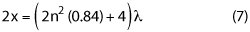Where the added 4 accounts for the 2lthickness of the absorber. If a different thickness of absorber is used, Equation 7 will change. In general, the chamber width can be written as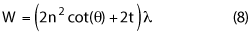Figure 6 Geometry of a far field range.

Parameters θ and t must be chosen to obtain the required reflectivity. It is important to keep a minimum 2λ spacing from the QZ to the absorber. The length of the rectangular far field chamber is mainly given by the far field distance and the QZ size plus the absorber thickness. Added space should be included for the range antenna and the absorber behind it.

The total chamber or range length (L) is given by: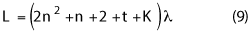where K is a factor large enough to include the source antenna, the 2λ spacing, and the absorber behind the source. It should be noted, that these equations provide a minimum requirement. Work must be performed inside the chamber — mounting and connecting the antenna, switching range antennas, etc. The space should be checked to allow for people to perform these tasks inside the anechoic range.

Expected chamber sizes can be examined by entering values into previous equations. It will be assumed that the source antenna is the directive with a sufficient front-to-back ratio. The absorber behind the source antenna will be one wavelength in thickness and the factor K will be set to 4.

In Figure 7, the width and length of a series of rectangular chambers have been plotted versus the lowest frequency of operation. In addition the electrical size of the AUT at the lowest frequency is indicated by the value of  n for each chamber size plotted.Figure 7 Rectangular far field chambers for different lowest frequencies of operation and different largest size antennas at their lowest frequencies.

If a chamber is designed for an antenna of a given nλ at the lowest frequency, that same chamber is large enough for testing antennas of the same electrical size at higher frequencies. Similarly, as the frequency is lowered, the chamber size must increase. At 500 MHz, a chamber for a 2λ sized antenna is about 10 × 5 m. If the antenna size was increased to 4λ, the chamber would need to be 18 × 10 m. Tapered anechoic chambers should be used at these lower frequencies.1,10-12 The geometry of the taper chamber uses the specular reflection off the side walls for the AUT illumination rather than reducing its level as it is done in the rectangular chamber. This leads to a physically smaller chamber.

The height of the chamber should be the same as the width. By doing this, the reflections from the ceiling and floor will be similar in level. This is important since the reflected energy from the ceiling and floor will be similar, and the effects of the range on polarization dependant parameters such as cross polarization and axial ratio will be minimized.

Equations 8 and 9 provide a good idea of the space requirements for an indoor range. In most cases, a chamber size can be adjusted. For example, the absorber on the ceiling and floor can be increased in thickness to maintain the reflectivity at more oblique angles of incidence (larger θ). Chebyshev arrangements13 of the absorber layout can also be used to improve reflectivity.

Figure 3 also reveals another clue to improve reflectivity. The reflected ray arrives at the range antenna at an angle at which the gain of the antenna is lower than in the boresight direction. Using higher directivity antennas as sources reduces the amount of energy received from the side walls, ceiling and floor. Hence, shorter absorbers reduce the chamber size.

Conclusion

Part one of this two-part series has dealt mainly with approximations for bi-static reflectivity of RF absorbers and the rectangular design of rectangular RF anechoic chambers. The polynomial equations include a “margin of safety” in their results. This helps in accounting for secondary bounces and edge diffractions as well as light fixtures, vents, doors and other disruptions of the absorber treatment. Part two will provide equations for compact ranges and near field to far field ranges.

Acknowledgment

The author will like to thank Zhong Chen for providing the computed results based on the NIST algorithm.8

References

1. L. Hemming, “Electromagnetic Anechoic Chambers: A Fundamental Design and Specification Guide,” IEEE Press/Wiley Interscience: Piscataway, N.J., 2002.
2. G. Sanchez and P. Connor, “How Much is a dB Worth?” 23rd Annual Symposium of the Antenna Measurement Techniques Association (AMTA), Denver, Colo., October 2001.
3. J. Hansen and V. Rodriguez, “Evaluate Antenna Measurement Methods,” Microwave and RF, October 2010, pp. 62–67.
4. W. Stutzma and G Thiele, “Antenna Theory and Design,” 2nd Ed., Wiley 1997 ANSI/IEEE STD 149-1979 149-1979 - IEEE Standard Test Procedures for Antennas, 1979, reaffirmed 2008.
5. D. Wayne, J. Fordham and J. McKenna, “Effects of a Non-Ideal Plane Wave on Compact Range Measurements,” 36th Annual Antenna Measurement Techniques Association Symposium (AMTA), Tucson, Ariz., October 2014.
6. V. Rodriguez, G. d’Abreu and K. Liu, “Measurements of the Power Handling of RF Absorber Materials: Creation of a Medium Power Absorber by Mechanical Means,” 31st Annual Antenna Measurement Techniques Association Symposium (AMTA), Salt Lake City, Utah, October 2009.
7. W. Sun and C. Balanis, “Analysis and Design of Periodic Absorbers by Finite-Difference Frequency-Domain Method,” Report No. TRC-EM-WS-9301 Telecommunications Research Center, Arizona State University, Tempe, Ariz., 1993.
8. E. Kuester and C. Holloway, “A Low-Frequency Model for Wedge or Pyramid Absorber Arrays-I: Theory,” IEEE Transactions on Electromagnetic Compatibility, Vol. 36, No. 4, November 1994.
9. V. Rodriguez, “A Study of the Effect of Placing Microwave Pyramidal Absorber on Top of Ferrite Tile Absorber on the Performance of the Ferrite Absorber,” 19th Annual Review of Progress in Computational Electromagnetics (ACES) Symposium, Monterey, Calif., March 2003.
10. W. Emerson and H. Sefton, “An Improved Design for Indoor Ranges,” Proceedings of the IEEE, Vol. 53, No. 8, pp. 107921081, 1965.
11. H. King, F. Shiukuro and J. Wong, “Characteristics of a Tapered Anechoic Chamber,” IEEE Transactions on Antennas Propagation, Vol. 15, No. 3, pp. 4882490, 1967.
12. V. Rodriguez, “Using Tapered Chambers to Test Antennas,” Evaluation Engineering, Vol. 43, No. 5, pp. 62268, 2004.
13. J-R J. Gau, D. Burnside and  M. Gilreath, “Chebyshev Multilevel Absorber Design Concept,” IEEE Transactions on Antennas Propagation, Vol. 45, No. 8, pp. 128621293, 1997.

Vince Rodriguez attended the University of Mississippi, in Oxford, Mississippi, where he obtained his B.S.E.E. in 1994. Following graduation, Rodriguez joined the department of Electrical Engineering at the University of Mississippi as a research assistant. During that time, he earned his M.S. and Ph.D. (both degrees in engineering science with emphasis in electromagnetics) in 1996 and 1999 respectively. Rodriguez joined EMC Test Systems (now ETS-Lindgren) as an RF and Electromagnetics engineer in 2000. He was the principal RF engineer for the anechoic chamber at the Brazilian Institute for Space Research (INPE), the largest chamber in Latin America and the only fully automatic, EMC and satellite testing chamber. In November 2014, Rodriguez joined MI Technologies in Suwanee, Ga. as a senior applications engineer bringing his expertise on numerical modeling, RF absorber and anechoic range design to the development of solutions for antenna, RCS and radome testing facility design.

Rodriguez is the author of more than 50 publications, including journal and conference papers as well as book chapters. He holds patents for a hybrid RF absorber and a dual ridge horn antenna. Rodriguez is a senior member of the IEEE and several of its technical societies. Among the IEEE technical societies, he is a member of the EMC Society, where he served as distinguished lecturer from 2012 to 2014 and also serves as member of the board of directors. He is an Edmund S. Gillespie Fellow of the Antenna Measurements Techniques Association (AMTA). Rodriguez is a member of the Applied Computational Electromagnetic Society (ACES), where he serves on the board of directors. Rodriguez is a member of several standard committees including IEEE STD 149, IEEE STD 1148 and RTCA DO-213. Rodriguez is also a full member of the Sigma Xi Scientific Research and Eta Kappa Nu Honor Societies.